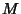## Manifold

Rigorously, an-D (topological) manifold is a Topological Spacesuch that any point inhas a Neighborhoodwhich is Homeomorphic to-D Euclidean Space. The Homeomorphism is called a chart, since it lays that part of the manifold out flat, like charts of regions of the Earth. So a preferable statement is that any object which can be ``charted'' is a manifold.

The most important manifolds are Differentiable Manifolds. These are manifolds where overlapping charts ``relate smoothly'' to each other, meaning that the inverse of one followed by the other is an infinitely differentiable map from Euclidean Space to itself.

Manifolds arise naturally in a variety of mathematical and physical applications as ``global objects.'' For example, in order to precisely describe all the configurations of a robot arm or all the possible positions and momenta of a rocket, an object is needed to store all of these parameters. The objects that crop up are manifolds. From the geometric perspective, manifolds represent the profound idea having to do with global versus local properties.

Consider the ancient belief that the Earthwas flat compared to the modern evidence that it is round. The discrepancy arises essentially from the fact that on the small scales that we see, the Earth does look flat. We cannot see it curve because we are too small (although the Greeks did notice that the last part of a ship to disappear over the horizon was the mast). We can detect curvature only indirectly from our vantage point on the Earth. The basic idea for this ``problem'' was codified by Poincaré.The problem is that on a small scale, the Earth is nearly flat. In general, any object which is nearly ``flat'' on small scales is a manifold, and so manifolds constitute a generalization of objects we could live on in which we would encounter the round/flat Earth problem.

See also Cobordant Manifold, Compact Manifold, Connected Sum Decomposition, Differentiable Manifold, Flag Manifold, Grassmann Manifold, Heegaard Splitting, Isospectral Manifolds, Jaco-Shalen-Johannson Torus Decomposition, Kähler Manifold, Poincaré Conjecture, Poisson Manifold, Prime Manifold, Riemannian Manifold, Set, Smooth Manifold, Space, Stiefel Manifold, Stratified Manifold, Submanifold, Surgery, Symplectic Manifold, Thurston's Geometrization Conjecture, Topological Manifold, Topological Space, Whitehead Manifold, Wiedersehen Manifold

References

Conlon, L. Differentiable Manifolds: A First Course. Boston, MA: Birkhäuser, 1993.